## Wednesday, May 10, 2023

### Preserving node labels in a plotted `cophylo` tanglegram

A phytools user has recently observed that plots generated via `phytools::cophylo` do not necessarily preserve the node indexing of the input tree.

I have posted about this earlier, although I have to apologize that the solution is a tiny bit messy.

Here’s a more complete worked example.

To start let’s load phytools and phangorn, then use the phangorn dataset called `"Laurasiatherian"`, and two different inference methods, to generate two different, potentially topologically incongruent trees.

``````## load packages
library(phytools)
``````
``````## Loading required package: ape
``````
``````## Loading required package: maps
``````
``````library(phangorn)
``````
``````## load dataset
data(Laurasiatherian)
``````

To start, we can obtain an MP tree using `phangorn::pratchet`.

``````## estimate tree using Maximum Parsimony
mp_tree<-pratchet(Laurasiatherian,trace=0)
mp_tree
``````
``````##
## Phylogenetic tree with 47 tips and 45 internal nodes.
##
## Tip labels:
##   Platypus, Wallaroo, Possum, Bandicoot, Opposum, Rabbit, ...
## Node labels:
##   1, 1, 0.89, 0.38, 0.39, 0.61, ...
##
## Unrooted; no branch lengths.
``````

Let’s go ahead & root our inferred tree using monotremes (`"Platypus"` in our phylogeny) as the outgroup.

``````rooted.mp_tree<-root(mp_tree,"Platypus",resolve.root=TRUE)
rooted.mp_tree
``````
``````##
## Phylogenetic tree with 47 tips and 46 internal nodes.
##
## Tip labels:
##   Platypus, Wallaroo, Possum, Bandicoot, Opposum, Rabbit, ...
## Node labels:
##   Root, 1, 1, 0.89, 0.38, 0.39, ...
##
## Rooted; no branch lengths.
``````

The `pratchet` method uses bootstrap sampling implicitly, and the bootstrap proportions are stored as node labels in our `"phylo"` object, so let’s plot our tree with node labels giving the bootstrap proportions of each clade as follows.

``````plotTree(rooted.mp_tree,nodes="centered",lwd=1,type="cladogram",
fsize=0.7)
nodelabels(rooted.mp_tree\$node.label[2:rooted.mp_tree\$Nnode],
2:rooted.mp_tree\$Nnode+Ntip(rooted.mp_tree),frame="none",
``````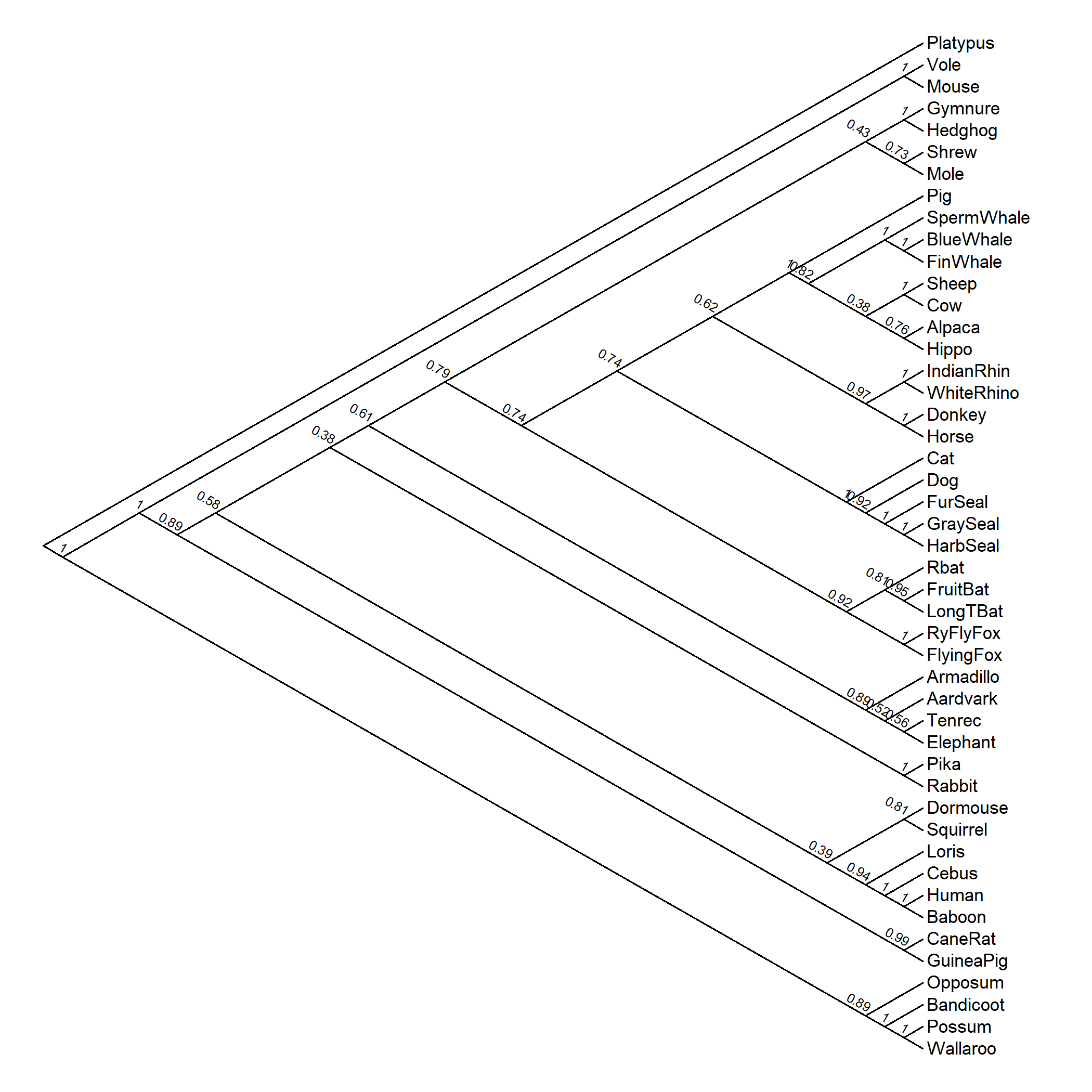OK, it’s not the prettiest graph I’ve ever seen, but you get the idea.

Now let’s do the same using ML.

``````ml_tree<-pml_bb(Laurasiatherian,model=" GTR+G(4)",
control=pml.control(trace=0))
``````

We’ll root our tree as before.

``````rooted.ml_tree<-root(ml_tree\$tree,"Platypus",resolve.root=TRUE)
``````

Now graph our bootstrap node labels on the tree.

``````plotTree(rooted.ml_tree,nodes="centered",lwd=1,
fsize=0.7)
nodelabels(rooted.ml_tree\$node.label[2:rooted.ml_tree\$Nnode],
2:rooted.ml_tree\$Nnode+Ntip(rooted.ml_tree),frame="none",
``````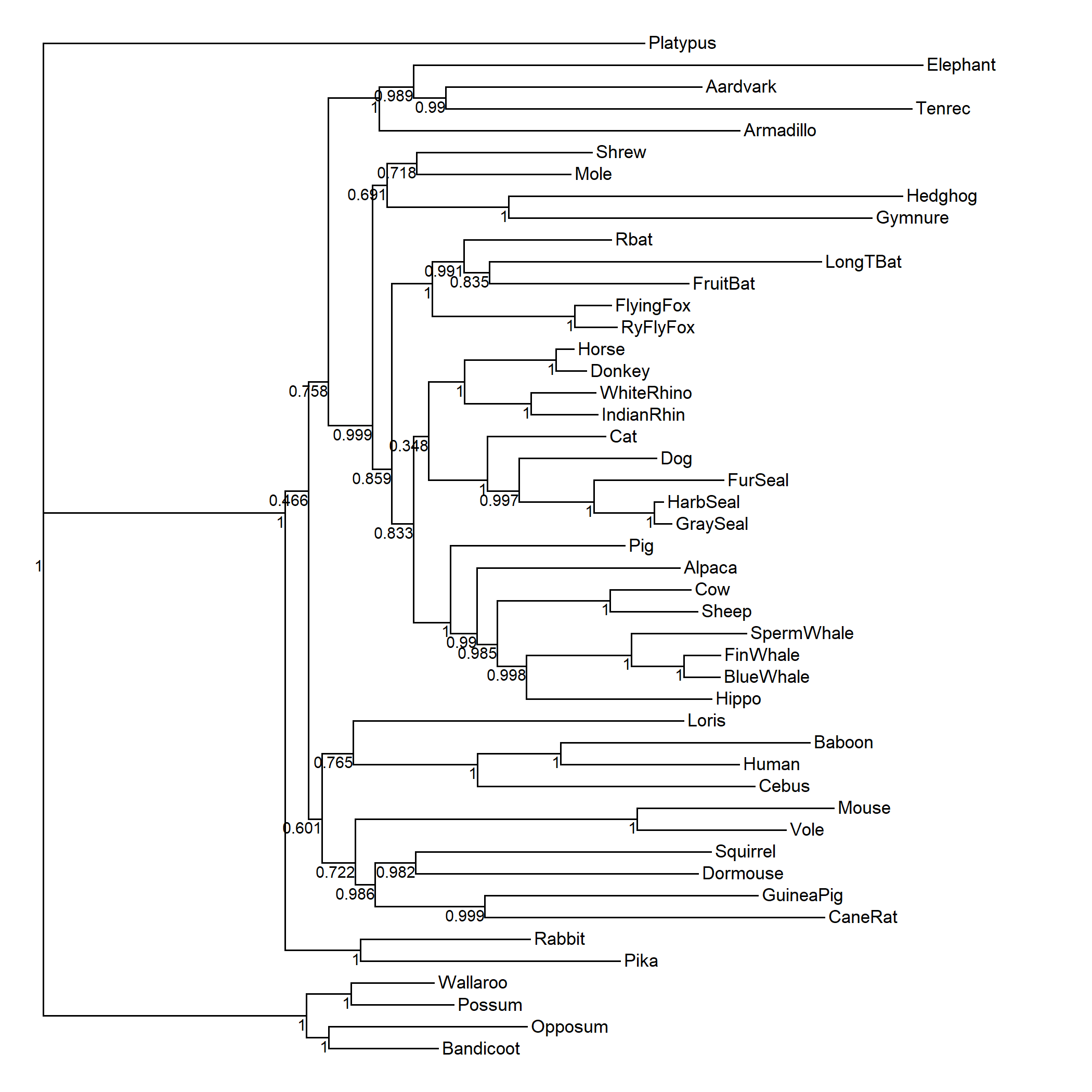These values, along with their clade associations, will be our point of reference in the tanglegram plot.

OK. We’re ready to compute our `"cophylo"` object!

``````obj<-cophylo(rooted.mp_tree,rooted.ml_tree)
``````
``````## Rotating nodes to optimize matching...
## Done.
``````

First, let’s graph it without node or edge labels.

(The option that I’m showing here, `type=c("cladogram","phylogram")`, will only work if you update phytools from GitHub.)

``````plot(obj,type=c("cladogram","phylogram"),fsize=0.6,part=0.48)
``````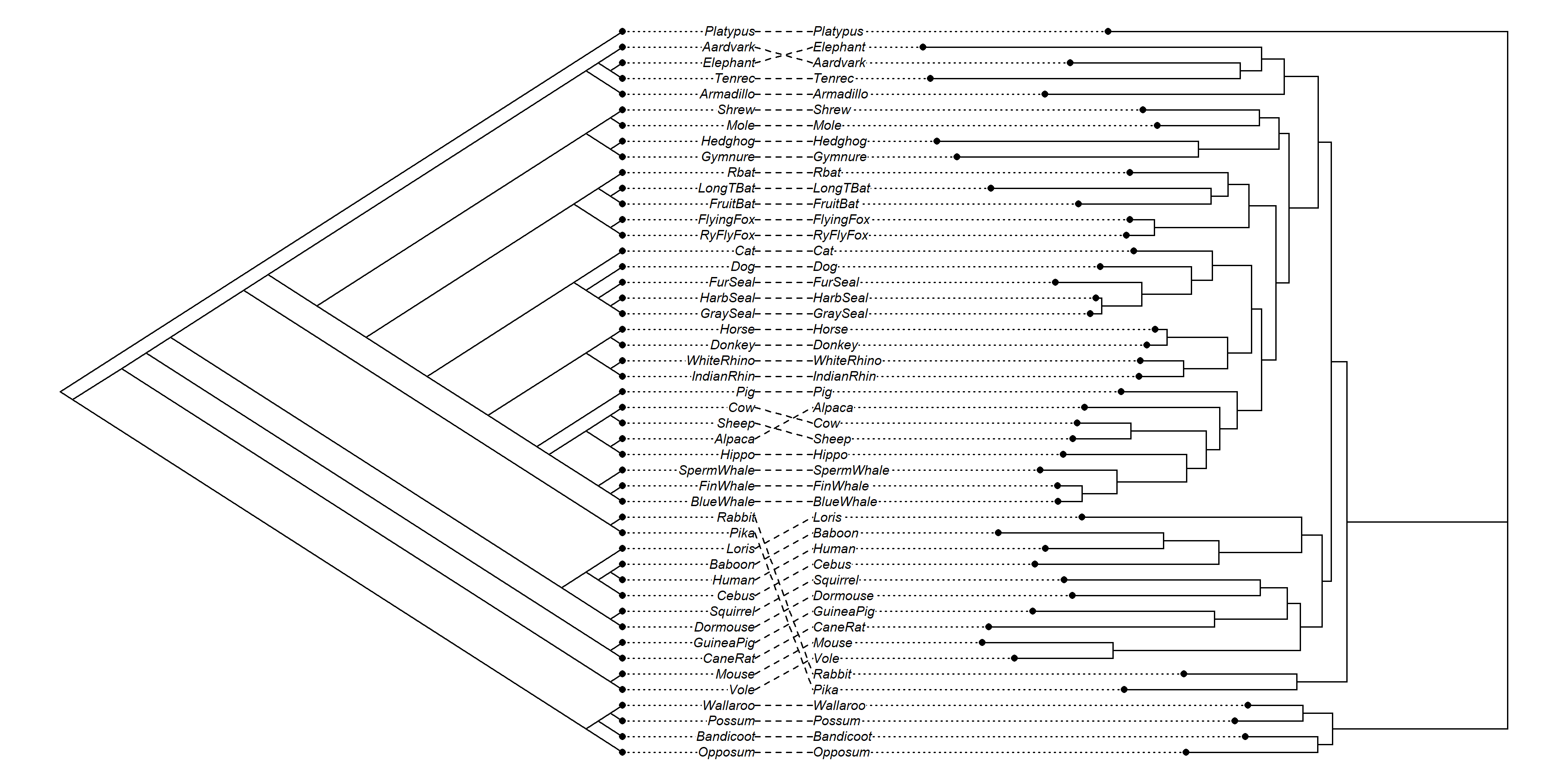Now let’s add in the node labels of our original `"phylo"` objects as in my previously given solution.

``````plot(obj,type="phylogram",fsize=0.6,part=0.48,
nodelabels.cophylo(obj\$trees[]\$node.label[2:Nnode(obj\$trees[])],
2:Nnode(obj\$trees[])+Ntip(obj\$trees[]),frame="none",
nodelabels.cophylo(obj\$trees[]\$node.label[2:Nnode(obj\$trees[])],
2:Nnode(obj\$trees[])+Ntip(obj\$trees[]),frame="none",
``````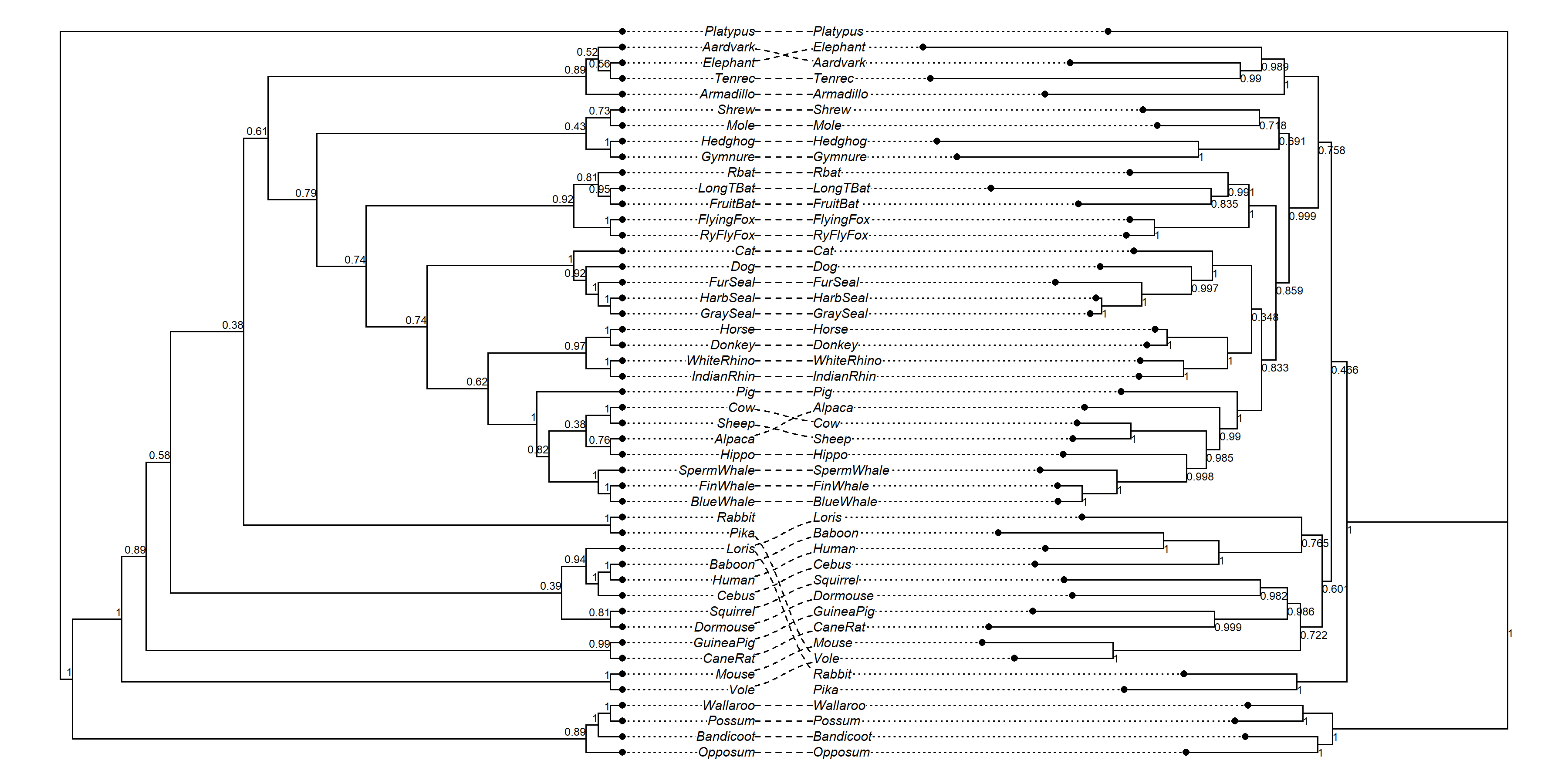Look closely at the values shown here compared to our earlier plots. Hopefully they match! (They’re meant to.)

Lastly, bootstrap proportions are usually represented as % values (i.e., between 0 and 100 instead of 0 and 1), and along edges, rather than at nodes.

Fortunately, we can do this too. Here’s one solution.

``````plot(obj,type="phylogram",fsize=0.6,part=0.48,
bs<-sapply(obj\$trees[]\$edge[,2]-Ntip(obj\$trees[]),
function(x,y) if(x>=2) as.numeric(y[x])*100 else "",
y=obj\$trees[]\$node.label)
which="left")
bs<-sapply(obj\$trees[]\$edge[,2]-Ntip(obj\$trees[]),
function(x,y) if(x>=2) as.numeric(y[x])*100 else "",
y=obj\$trees[]\$node.label)
which="right")
``````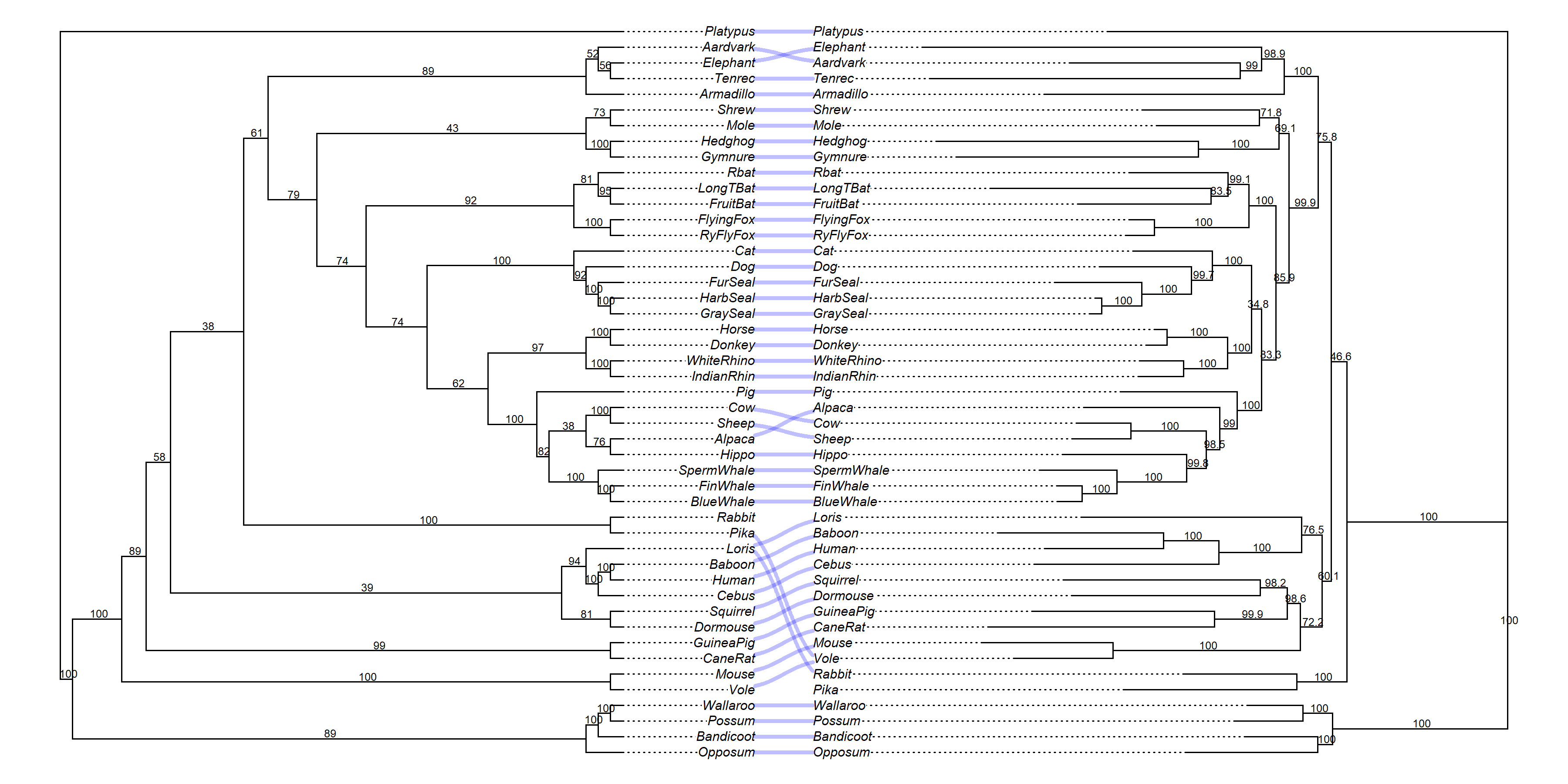Right?

OK. I’ll think about how to make this possible to do with a bit less scripting!

That’s about all I can handle for now, though!